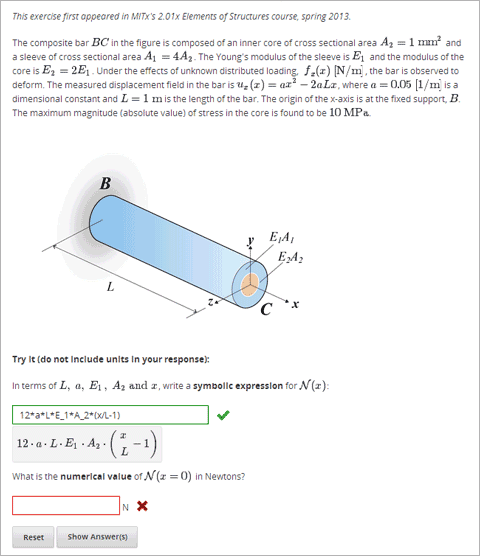# 7.19. Math Expression Input Problems¶

In math expression input problems, learners enter text that represents a mathematical expression. The text is converted to a symbolic expression that appears below the response field. Unlike numerical input problems, which only allow integers and a few select constants, math expression input problems can include unknown variables and more complicated symbolic expressions.For the math expression input problems in your course, you can use edX Insights to review aggregated learner performance data and examine submitted answers. For more information, see Using edX Insights.

For math expression input problems, the grader uses numerical sampling to determine whether a learner’s response matches the math expression that you provide, to a specified numerical tolerance. You specify the allowed variables in the expression as well as the range of values for each variable.

When you create a math expression input problem in Studio, you use MathJax to change your plain text into “beautiful math.” For more information about how to use MathJax in Studio, see A Brief Introduction to MathJax in Studio.

Note

Math expression input problems currently cannot include negative numbers raised to fractional powers, such as (-1)^(1/2). Math expression input problems can include complex numbers raised to fractional powers, or positive non-complex numbers raised to fractional powers.

## 7.19.1. Create a Math Expression Input Problem¶

To create a math expression input problem, follow these steps.

1. In the unit where you want to create the problem, under Add New Component select Problem.
3. Select Math Expression Input. Studio adds an example math expression input problem to the unit.
4. Select Edit. The Advanced Editor opens.
5. Replace the sample problem XML with your own marked up text. To practice, you can use the example problem that follows.
6. Select Settings and provide an identifying Display Name for the problem.
8. Select Save.

Example Problem Code

<problem>
<p>Some problems may ask for a mathematical expression. Practice creating mathematical expressions by answering the questions below.</p>

<p>Write an expression for the product of R_1, R_2, and the inverse of R_3.</p>
<formularesponse type="ci" samples="R_1,R_2,R_3@1,2,3:3,4,5#10" answer="$VoVi"> <responseparam type="tolerance" default="0.00001"/> <formulaequationinput size="40" label="Enter the equation"/> </formularesponse> <script type="loncapa/python"> VoVi = "(R_1*R_2)/R_3" </script> <p>Let <i>x</i> be a variable, and let <i>n</i> be an arbitrary constant. What is the derivative of <i>x<sup>n</sup></i>?</p> <script type="loncapa/python"> derivative = "n*x^(n-1)" </script> <formularesponse type="ci" samples="x,n@1,2:3,4#10" answer="$derivative">
<responseparam type="tolerance" default="0.00001"/>
<formulaequationinput size="40"  label="Enter the equation"/>
</formularesponse>

<solution>
<div class="detailed-solution">
<p>Explanation or solution text</p>
</div>
</solution>
</problem>


## 7.19.2. Math Expression Input Problem XML¶

### 7.19.2.1. Templates¶

<problem>
<p>Write an expression for the product of R_1, R_2, and the inverse of R_3.</p>
<responseparam type="tolerance" default="0.00001"/>
<formulaequationinput size="40"  label="Enter the equation" />
</formularesponse>
</problem>

<problem>
<p>Problem text</p>
<formularesponse type="ci" samples="VARIABLES@LOWER_BOUNDS:UPPER_BOUNDS#NUMBER_OF_SAMPLES" answer="$VoVi"> <responseparam type="tolerance" default="0.00001"/> <formulaequationinput size="20" label="Enter the equation" /> </formularesponse> <script type="loncapa/python"> PYTHON SCRIPT </script> <solution> <div class="detailed-solution"> <p>Explanation or Solution Header</p> <p>Explanation or solution text</p> </div> </solution> </problem>  ### 7.19.2.2. Tags¶ • <formularesponse> • <formulaequationinput /> • <responseparam> • <script> Tag: <formularesponse> Specifies that the problem is a math expression input problem. The <formularesponse> tag is similar to <numericalresponse>, but <formularesponse> allows unknown variables. Attributes type: Can be “cs” for case sensitive, which is the default, or “ci” for case insensitive, so that capitalization does not matter in variable names. answer: The correct answer to the problem, given as a mathematical expression. If you precede a variable name in the problem with a dollar sign ($), you can include a script in the problem that computes the expression in terms of that variable.

samples: Specifies important information about the problem in the following lists.

• variables: A set of variables that learners can enter.
• lower_bounds: For every defined variable, a lower bound on the numerical tests to use for that variable.
• upper_bounds: For every defined variable, an upper bound on the numerical tests to use for that variable.
• num_samples: The number of times to test the expression.

Commas separate items inside each of the four individual lists. The at sign (@), colon (:), and pound sign (#) characters separate the lists. An example of the format follows.

"variables@lower_bounds:upper_bounds#num_samples"

For example, a <formularesponse> tag that includes the samples attribute might look like either of the following.

<formularesponse samples="x,n@1,2:3,4#10">

<formularesponse samples="R_1,R_2,R_3@1,2,3:3,4,5#10">

Children

<formulaequationinput />

Tag: <formulaequationinput />

Creates a response field where a learner enters an answer to the problem in plain text, as well as a second field below the response field where the learner sees a typeset version of the plain text. The parser that renders the learner’s plain text into typeset math is the same parser that evaluates the learner’s response for grading.

Attributes

 Attribute Description label (required) Specifies the name of the response field. size (optional) Specifies the width, in characters, of the response field where learners enter answers.

Children

(none)

Tag: <responseparam>

Used to define an upper bound on the variance of the numerical methods used to approximate a test for equality.

Attributes

 Attribute Description default (required) A number or a percentage specifying how close the learner and grader expressions must be. If you do not include a tolerance, the expression is vulnerable to rounding errors during sampling. The result of such unavoidable errors is that the grader can mark some learner input as incorrect, even if it is algebraically equivalent. type “tolerance”, which defines a tolerance for a number.

Children

(none)